# php数组里面可放数组吗

php数组里面可以放数组。PHP数组可以存储所有类型的数据，当然也包括数组本身；如果一个数组中的元素是另一个数组，就构成了包含数组的数组，即多维度数组。数组的不同维度标志着需用几个下标（索引）来获取对应的数组元素，比如二维数组需用两个下标。php数组里面可以放数组。

PHP 中的数组可以存储所有类型的数据，当然也包括数组本身。如果一个数组中的元素是另一个数组，就构成了包含数组的数组，即多维度数组：

• 二维数组

• 三维数组

• 四维数组

• .....

1、定义二维数组

1）、直接为数组元素赋值

```<?php
\$array['姓名'] = '张三';
\$array['年龄'] = '25';
\$array['性别'] = '男';
\$array['姓名'] = '李四';
\$array['年龄'] = '21';
\$array['性别'] = '男';
\$array['姓名'] = '娜娜';
\$array['年龄'] = '22';
\$array['性别'] = '女';
var_dump(\$array);
?>```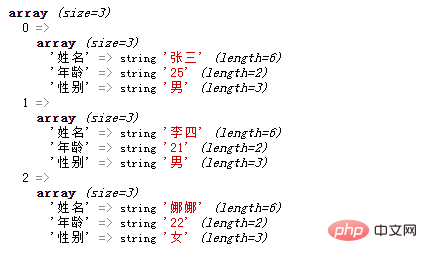```<?php
\$array[] = '张三';
\$array[] = '25';
\$array[] = '男';
\$array[] = '李四';
\$array[] = '21';
\$array[] = '男';
\$array[] = '娜娜';
\$array[] = '22';
\$array[] = '女';
var_dump(\$array);
?>```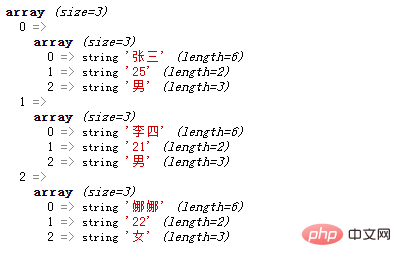```<?php
\$array[]['姓名'] = '张三';
\$array[]['年龄'] = '25';
\$array[]['性别'] = '男';
\$array[]['姓名'] = '李四';
\$array[]['年龄'] = '21';
\$array[]['性别'] = '男';
\$array[]['姓名'] = '娜娜';
\$array[]['年龄'] = '22';
\$array[]['性别'] = '女';
var_dump(\$array);
?>```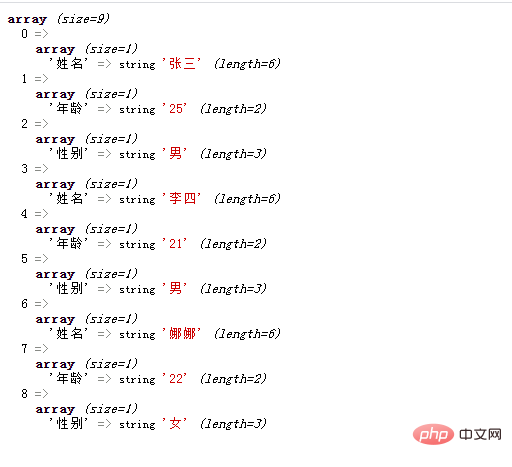```<?php
\$array[][] = '张三';
\$array[][] = '25';
\$array[][] = '男';
\$array[][] = '李四';
\$array[][] = '21';
\$array[][] = '男';
\$array[][] = '娜娜';
\$array[][] = '22';
\$array[][] = '女';
var_dump(\$array);
?>```2）、使用array()函数

• 索引数组

```<?php
\$array = array
(
array("张三",25,"男"),
array("李四",21,"男"),
array("娜娜",22,"女")
);
var_dump(\$array);
?>```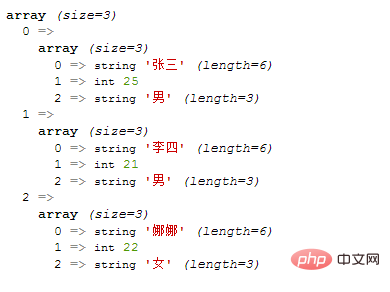• 关联数组

```<?php
\$array = array
(
"学生1"=>array("张三",25,"男"),
"学生2"=>array("李四",21,"男"),
"学生3"=>array("娜娜",22,"女")
);
var_dump(\$array);
?>```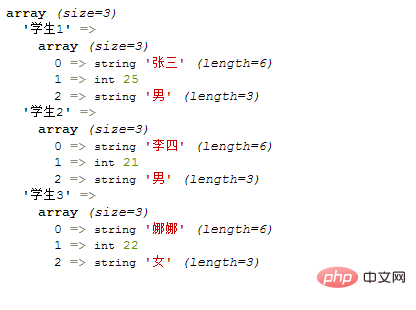```<?php
\$array = array
(
"学生1"=>array("姓名"=>"张三","年龄"=>25,"性别"=>"男"),
"学生2"=>array("姓名"=>"李四","年龄"=>21,"性别"=>"男"),
"学生3"=>array("姓名"=>"娜娜","年龄"=>22,"性别"=>"女")
);
var_dump(\$array);
?>```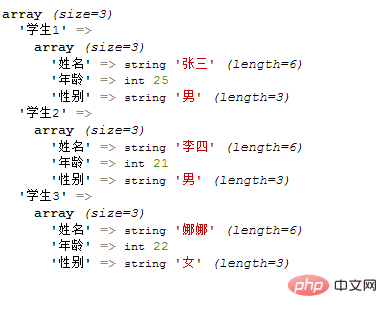```<?php
\$array = array
(
array("姓名"=>"张三","年龄"=>25,"性别"=>"男"),
array("姓名"=>"李四","年龄"=>21,"性别"=>"男"),
array("姓名"=>"娜娜","年龄"=>22,"性别"=>"女")
);
echo \$array['姓名'].'同学的年龄为'.\$array['年龄'].'岁';
?>```2、多维数组的创建

```<?php
\$array = array(
'安徽' => array(
'合肥'=>array('蜀山区','长丰县','肥东'),
'宿州'=>array('墉桥区','灵璧县','泗县')
),
'河南' => array(
'洛阳'=>array('西工区','老城区','孟津县'),
'郑州市'=>array('中原区','金水区')
)
);
var_dump(\$array);
?>```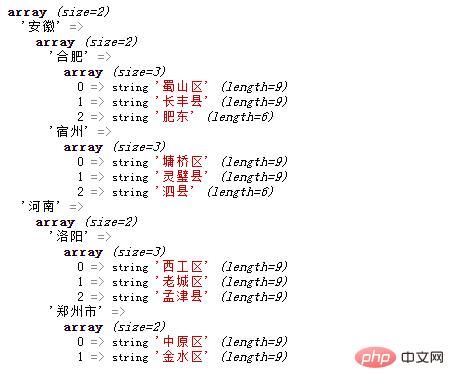1/1